Question

# Consider a discrete-time LTI system with impulse response hn on-un-1), where jal < 1. Find the...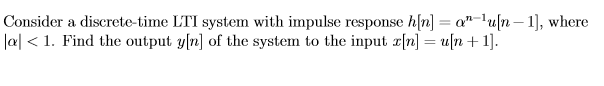Consider a discrete-time LTI system with impulse response hn on-un-1), where jal < 1. Find the output y[n] of the system to the input x[n] = un +1].

We need at least 10 more requests to produce the answer.

0 / 10 have requested this problem solution

The more requests, the faster the answer.

All students who have requested the answer will be notified once they are available.

#### Earn Coins

Coins can be redeemed for fabulous gifts.

Similar Homework Help Questions
• ### CONVOLUTION - Questions 4 and 5 4. Consider an LTI system with an impulse response h(n)...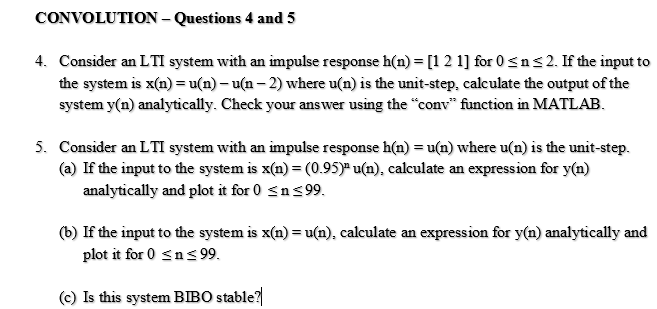CONVOLUTION - Questions 4 and 5 4. Consider an LTI system with an impulse response h(n) = [1 2 1] for 0 <n<2. If the input to the system is x(n) = u(n)-un-2) where u(n) is the unit-step, calculate the output of the system y(n) analytically. Check your answer using the "conv" function in MATLAB. 5. Consider an LTI system with an impulse response h(n) = u(n) where u(n) is the unit-step. (a) If the input to the system is...

• ### Problem 2 Consider a continuous-time LTI system whose frequency response is given by 2 sin(40 (a)...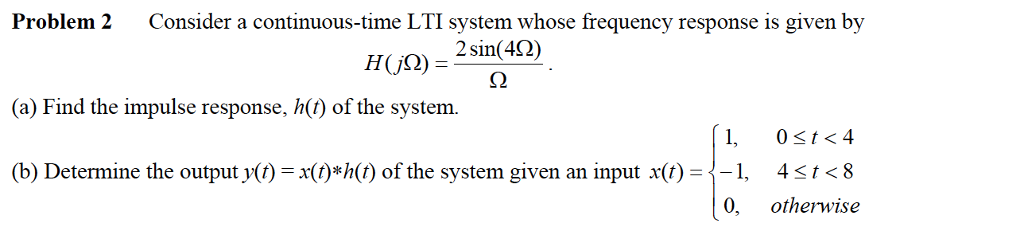Problem 2 Consider a continuous-time LTI system whose frequency response is given by 2 sin(40 (a) Find the impulse response, h(t) of the system (b) Determine the outputy() =x(t)*h(t) of the system given an input x(f)--1, ț < 8 4 otherwise 0,

• ### 6) Consider a discrete-time LTI system with impulse response h[n] = response h[n] = ( 1)...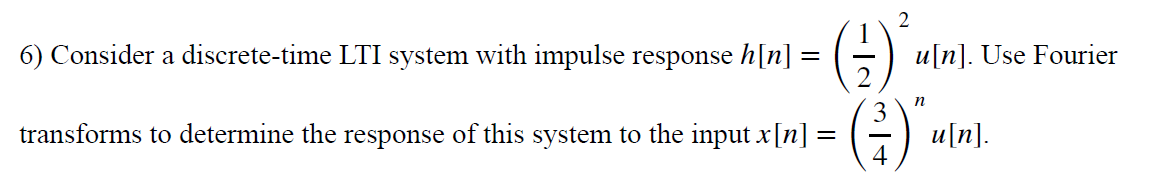6) Consider a discrete-time LTI system with impulse response h[n] = response h[n] = ( 1) u[n]. Use Fourie transforms to determine the response of this system to the input x[n] = ml + un).

• ### 1. Convolution of Discrete Time Signals Analytically determine the convolutions: (a) Find yn]-nun], where un] is...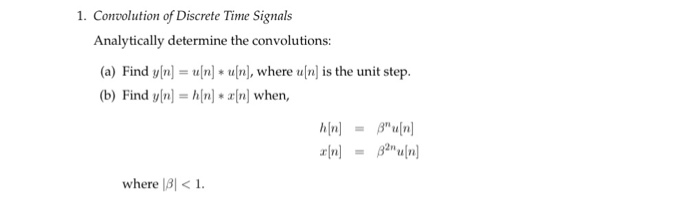1. Convolution of Discrete Time Signals Analytically determine the convolutions: (a) Find yn]-nun], where un] is the unit step (b) Find yln] z[n] when, where 131 <1

• ### Consider an LTI discrete-time system that has impulse response h n Tn-12) 1 if otherwise a) Deter...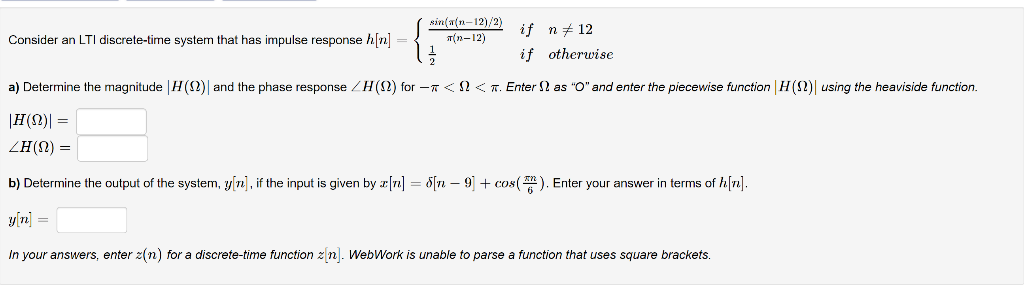Consider an LTI discrete-time system that has impulse response h n Tn-12) 1 if otherwise a) Determine the magnitude H(Q and the phase response LH(D for-r < Ω < π Enter Ω as "and enter the piecev se function Η Ω using the hea side function b)Determine the output of the system, rn, if the input is given by z n-Sn-9 +com( ) Enter your answer in terms of hin y[n] = In your answers, enter 2(n) for a discrete-time...

• ### Problem 1 You are given the discrete-time LTI system with impulse response, Calculate the Fourier series coefficients o...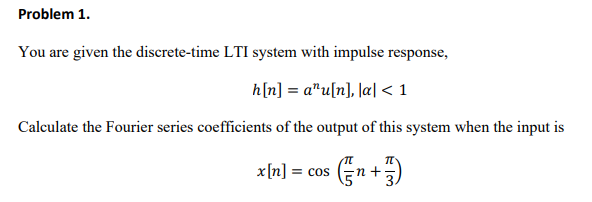Problem 1 You are given the discrete-time LTI system with impulse response, Calculate the Fourier series coefficients of the output of this system when the input is x[n] = cos(n+π) Problem 1 You are given the discrete-time LTI system with impulse response, Calculate the Fourier series coefficients of the output of this system when the input is x[n] = cos(n+π)

• ### BC:9.4 A LTI discrete time system has an impulse response h[n] = (-0.8)"u[n] + (0.65)"u[n-1] Find...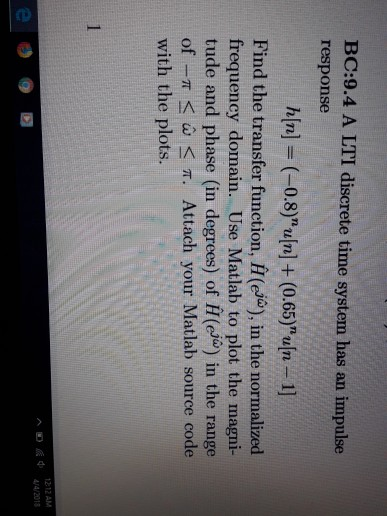BC:9.4 A LTI discrete time system has an impulse response h[n] = (-0.8)"u[n] + (0.65)"u[n-1] Find the transfer function, #(eo), in the normalized frequency domain. Use Matlab to plot the magni- tude and phase (in degrees) of H(eo) in the range of-? < ? < ?. Attach your Matlab source code with the plots. 1212 AM ^???4/4/2013

• ### 4. Consider the magnitude and phase of the frequency response Hi(2) of a linear and time-invariant...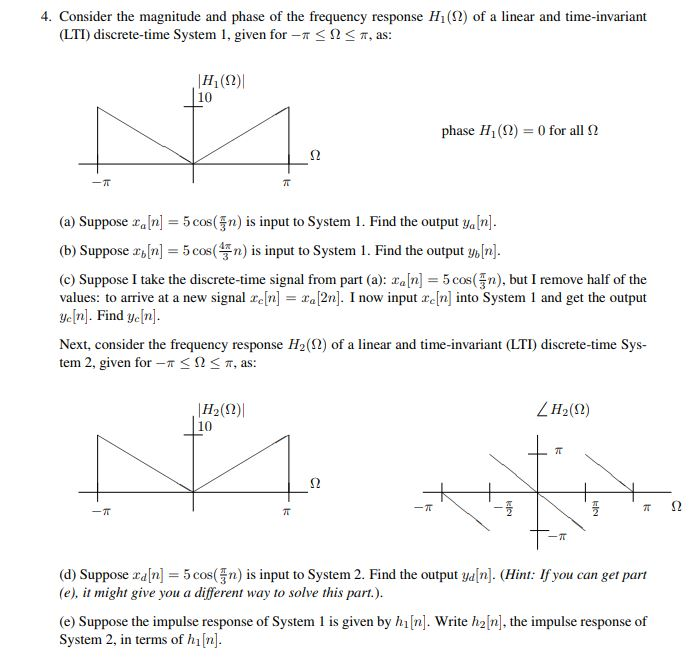4. Consider the magnitude and phase of the frequency response Hi(2) of a linear and time-invariant (LTI) discrete-time System 1, given for-r < Ω-T, as: H, (12)| 10 phase H1(Ω)--0 for all Ω (a) Suppose an 5cos(n s input to System 1. Find the output ya[n] (b) Suppose ancos(is input to System 1. Find the output ybn] (c) Suppose I take the discrete-time signal from part (a): xa[n] 5cos(n), but I remove half of the values: to arrive at a...

• ### Discrete-time convolution. Use of shift invariance for LTI systems. A discrete-time LTI system is described the...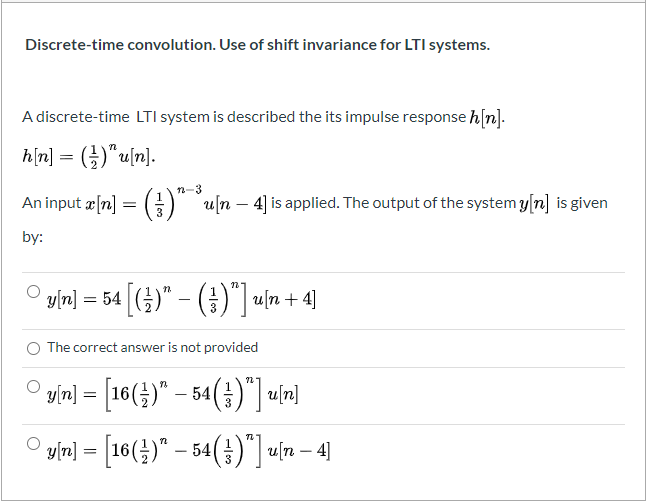Discrete-time convolution. Use of shift invariance for LTI systems. A discrete-time LTI system is described the its impulse response h[n]. h[n] = (5)"u[n]. n-3 1 An input x[n] = u[n – 4) is applied. The output of the system y[n] is given by: x[r] – 54 G)" ()") un 14 The correct answer is not provided gắn] = [16(5)” – 54(5) ] n] y[n] = [16()" – 54(+)"] uſn – 4

• ### Consider a discrete-time LTI system with impulse response Sketch the magnitude of the frequency response of...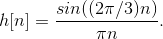Consider a discrete-time LTI system with impulse response Sketch the magnitude of the frequency response of the system. Provide enough details in your sketch to convey the pattern. sin((2n/3)n hln h[n] =